• 反向传播是关于理解如何改变网络中的权重和偏差来改变成本函数。最终，这意味着计算偏导数 ∂C/∂wjkl\partial C/\partial w^l_{jk}∂C/∂wjkl​ 和 ∂C/∂bjl\partial C/\partial b^l_{j}∂C/∂bjl​ 。但为了计算...
反向传播
参考链接
反向传播是关于理解如何改变网络中的权重和偏差来改变成本函数。最终，这意味着计算偏导数 $\partial C/\partial w^l_{jk}$ 和 $\partial C/\partial b^l_{j}$ 。但为了计算这些，我们首先引入一个中间量 $\delta_j^l$ ，我们称其为第l层第j个神经元的误差。Backpropagation 将给我们一个计算误差 $\delta_j^l$ 的程序，然后将 $\delta_j^l$ 与 $\partial C/\partial w^l_{jk}$ 和 $\partial C/\partial b^l_{j}$ 相关联。
为了理解错误是如何定义的，想象我们的神经网络中有一个恶魔:恶魔坐在第l层的第j个神经元处，当神经元的输入进来时，恶魔就会扰乱神经元的运作。它在神经元的加权输入中增加了一点变化$Δz^l_j$，所以神经元不是输出$σ(z^l_j)$，而是输出$σ(z^l_j+Δz^l_j)$。这种变化会通过网络中的后几层传播，最后导致整体成本发生变化，变化量为$\frac {∂C}{∂z^l_j}Δz^l_j$。
现在，这个妖怪是个好妖怪，是想帮你改善成本函数，也就是说，他们是想找到一个能让成本函数变小的$Δz^l_j$。 假设$\frac {∂C}{∂z^l_j}$有一个很大的值（正或负）。那么恶魔可以通过选择$Δz^l_j$与$\frac {∂C}{∂z^l_j}$的相反符号来降低不少成本。相反，如果$\frac {∂C}{∂z^l_j}$接近于零，那么恶魔通过扰动加权输入$z^l_j$根本无法提高多少成本。就恶魔所能知道的，神经元已经很接近最优*了。所以有一个启发式的意义，$\frac {∂C}{∂z^l_j}$是对神经元的误差的衡量。
在这个故事的激励下，我们用以下方式定义层l中神经元j的误差$δ^l_j$按照我们的惯例，我们用 $δ^l$ 来表示与第l层相关的误差向量。反向传播将使我们有办法计算每一层的 $δ^l$ ，然后将这些误差与实际利益量$∂C/∂w^l_{jk}$和$∂C/∂b^l_j$联系起来。你可能会好奇为什么恶魔要改变加权输入$z^l_j$。当然，想象恶魔改变输出激活$a^l_j$会更自然一些，结果是我们会使用$\frac{∂C}{∂a^l_j}$作为我们的误差测量。
事实上，如果你这样做，事情的结果和下面的讨论很相似。但事实证明，这使得反传播的呈现方式在代数上更加复杂一些。所以我们将坚持用$δ^l_j=\frac{∂C}{∂z^l_j}$作为我们对误差的测量。
Plan of attack:  反向传播是基于四个基本方程。这些方程一起给我们提供了一种计算误差$δ_l$和成本函数梯度的方法。我在下面陈述这四个方程。但要注意：你不应该期望瞬间吸收这些方程。这样的期望会导致失望。事实上，反推方程的内容非常丰富，随着你对方程的逐步深入，理解好这些方程需要相当的时间和耐心。好消息是，这种耐心会得到多次回报。因此，本节的讨论只是一个开始，帮助你在彻底理解方程的路上。
这里是我们在本章后面更深入研究方程的方法的预览。我将给出方程的一个简短证明，这有助于解释为什么它们是真实的；我们将以伪代码的算法形式重述这些方程，并看看如何将伪代码作为真实的、正在运行的Python代码来实现；在本章的最后一节，我们将对反向传播方程的含义进行直观的描述，以及某人如何从头开始发现它们。一路走来，我们会反复回到四个基本方程，随着你对这些方程理解的加深，这些方程会显得很舒服，甚至可能是美丽而自然的。
An equation for the error in the output layer, $δ^L$:
$δ^L$ 的分量由以下公式给出这是一个非常自然的表达。右边的第一个项$∂C/∂a^L_j$只是衡量成本函数对第j个输出激活函数的变化速度。例如，如果C对某一特定输出神经元j的依赖性不大，那么$δ^L_j$就会很小，这是我们所期望的。右边第二个项，$σ′(z^L_j)$，衡量激活函数σ在$z^L_j$处变化的速度。
请注意，（BP1）中的所有内容都很容易计算。特别是，我们在前向时计算zLj，计算$σ′(z^L_j)$只是一个小小的额外开销。当然，$∂C/∂a^L_j$的确切形式将取决于成本函数的形式。然而，只要成本函数已知，计算$∂C/∂a^L_j$应该没有什么问题。例如，如果我们使用二次成本函数，那么$C=\frac{1}{2}∑_j(y_j-a^L_j)^2$，所以$∂C/∂a^L_j=(a^L_j-y_j)$，显然这很容易计算。
等式（BP1）是$δ^L$的分量表达式。这是一个非常好的表达式，但不是我们想要的基于矩阵的反推形式。然而，很容易将方程改写成基于矩阵的形式，如这里，$∇_aC$被定义为一个矢量，其分量为偏导数$∂C/∂a^L_j$。 您可以把$∇_aC$看作是C相对于输出激活的变化率。很容易看出，方程（BP1a）和（BP1）是等价的，出于这个原因，从现在开始，我们将交替使用（BP1）来指代这两个方程。举个例子，在quadratic cost的情况下，我们有$∇_aC=(a^L-y)$，因此(BP1)的完全矩阵形式变成了正如你所看到的，这个表达式中的所有内容都有一个漂亮的向量形式，并且很容易使用Numpy等库进行计算。
用下一层的误差$δ^{l+1}$来表示误差$δ^l$的公式：特别是这个公式看起来很复杂，但每个元素都有一个很好的解释。假设我们知道第l+1层的误差$δ^{l+1}$。 当我们应用转置权重矩阵$(w^{l+1})^T$时，我们可以直观地认为这是通过网络向后移动误差，给我们提供了第l层输出处误差的某种测量。
然后我们取Hadamard product $⊙σ′(z^l)$。这就将误差通过层l中的激活函数向后移动，得到层l的加权输入中的误差$δ^l$。
通过将(BP2)与(BP1)相结合，我们可以计算出网络中任何一层的误差$δ^l$。我们先用(BP1)计算出$δ^l$l，然后应用公式(BP2)计算出$δ^{l-1}{}$，再应用公式(BP2)计算出$δ^{l-2}$，以此类推。
关于网络中任何偏置的成本变化率的公式。特别是也就是说，误差$δ^l_j$正好等于变化率$∂C/∂b^l_j$。这是一个好消息，因为(BP1)和(BP2)已经告诉我们如何计算$δ^l_j$。我们可以将(BP3)简写为其中，可以理解为δ与偏置b在同一神经元处被评估。
成本相对于网络中任何权重的变化率的公式。特别是：这告诉我们如何用$δ^l$和$a^{l-1}$这两个量来计算部分导数$∂C/∂w^l_{jk}$，我们已经知道如何计算。该方程可以用一个less index-heavy notation重写为等式(32)的一个很好的结果是，当激活$a_{in}$很小，$a_{in}$≈0时，梯度项∂C/∂w也将非常小。在这种情况下，我们会说权重学习得很慢，也就是说在梯度下降过程中，权重变化不大。换句话说，（BP4）的一个结果是，低激活神经元输出的权重学习速度很慢。
从(BP1)-(BP4)中还可以按照这些思路得到其他的启示。让我们从观察输出层开始。考虑(BP1)中的 $σ′(z^L_j)$ 项。
从上一章的sigmoid函数图中回想一下，当$σ(z^L_j)$约为0或1时，σ函数会变得非常平坦。当这种情况发生时，我们将有$σ′(z^L_j)$ ≈0.所以教训是，如果输出神经元是低激活(≈0)或高激活(≈1)，最后一层的权重将缓慢学习。在这种情况下，通常说输出神经元已经饱和，因此，权重已经停止学习（或学习缓慢）。类似的说法也适用于输出神经元的偏差。
我们可以对更早的层获得类似的见解。特别是注意（BP2）中的$σ′(z^l)$项。这意味着，如果神经元接近饱和，$δ^l_j$很可能变得很小。而这又意味着输入到饱和神经元的任何权重都会学习缓慢。
综上所述，我们已经了解到，如果输入神经元是低激活，或者输出神经元已经饱和，即是高激活或者低激活，那么权重就会学习很慢。
这些观察都没有太大惊奇。不过，它们还是有助于改善我们对神经网络学习时的心理模型。此外，我们可以把这种类型的推理转过来。四个基本方程原来对任何激活函数都是成立的，而不仅仅是标准的sigmoid函数（这是因为，我们一会儿会看到，证明没有使用σ的任何特殊属性）。因此，我们可以利用这些方程来设计具有特定理想学习特性的激活函数。举个例子给大家讲讲，假设我们选择一个（非sigmoid）激活函数σ，使σ′总是正值，并且永远不会接近零。这样就可以防止普通的sigmoid神经元饱和时出现的学习减速。在本书的后面，我们将看到对激活函数进行这种修改的例子。牢记四个方程（BP1）-（BP4）可以帮助解释为什么要尝试这种修改，以及它们会产生什么影响。Problem
反推方程的交替呈现:
我已经用Hadamard乘积陈述了反推公式（特别是（BP1）和（BP2））。如果你不习惯使用Hadamard乘积，这种表述可能会让你感到不安。有一种基于传统矩阵乘法的替代方法，有些读者可能会觉得很有启发。(1)说明(BP1)可以改写为其中$Σ′(z^L)$是一个正方形矩阵，它的对角线的项是$σ′(z^L_j)$的值，它的非对角的项为零。请注意，该矩阵通过常规矩阵乘法作用于$∇_aC$。(2)说明(BP2)可改写为(3)结合观察(1)和(2)说明对于熟悉矩阵乘法的读者来说，这个公式可能比(BP1)和(BP2)更容易理解。我之所以把重点放在(BP1)和(BP2)上，是因为这种方法在数值上实现起来更快。
Proof of the four fundamental equations (optional)
现在我们将证明四个基本方程(BP1)-(BP4)。这四个方程都是多变量微积分中的链式规则的结果。如果你对链式规则很熟悉，那么我强烈鼓励你在继续阅读之前自己尝试推导。
让我们从等式（BP1）开始，它给出了输出误差的表达式$δ^L$。为了证明这个等式，请回顾一下，根据定义应用链式规则，我们可以将上面的偏导数重新表达为关于输出激活的偏导数。其中，和为输出层中所有神经元k的和。当然，当k=j时，第k个神经元的输出激活$a^L_k$只取决于第j个神经元的加权输入$z^L_j$。所以当k≠j时，$∂a^L_k/∂z^L_j$消失。因此，我们可以将上式简化为回顾$a^L_j=σ(zLj)$，右边的第二个项可以写成$σ′(z^L_j)$，方程就变成了接下来，我们将证明(BP2)，用下一层的误差$δ^{l+1}$给出误差$δ^l$的公式。为此，我们要将$δ^l_j=∂C/∂z^l_j$改写为$δ^{l+1}_k=∂C/∂^{zl+1}_k$。我们可以使用链式规则来实现：其中，在最后一行，我们将右侧的两个项互换，并代入$δ^{l+1}_k$的定义。为了评估最后一行的第一个项，请注意到以下几点微分后，我们得到将其代入(42)，我们得到我们要证明的最后两个方程是（BP3）和（BP4）。这两个方程也是由链式法则得出的，与上述两个方程的证明方式类似。
这就完成了反传的四个基本方程的证明。这个证明可能看起来很复杂。但其实这只是仔细应用链式规则的结果。稍微简洁一点的说，我们可以把反向传播看成是通过系统地应用多变量微积分中的链式规则来计算成本函数梯度的一种方法。这就是反向传播的全部内容，剩下的就是细节了。
The backpropagation algorithm
反推方程为我们提供了一种计算成本函数梯度的方法。让我们以算法的形式明确地写出来。

Input x：输入层相应的激活设置$a^1$。研究这个算法你就能明白为什么叫反向传播。我们从最后一层开始，向后计算错误向量$δ^l$。我们要向后穿过网络，这可能看起来很奇怪。但如果你思考一下反向传播的证明，向后移动是成本是网络输出的函数这一事实的结果。为了理解成本是如何随着早期的权重和偏差而变化的，我们需要反复应用链式规则，通过层层后移来获得可用的表达式。
In what sense is backpropagation a fast algorithm?
在什么意义上，反向传播是一种快速算法？为了回答这个问题，我们来考虑另一种计算梯度的方法。想象一下，现在是神经网络研究的早期。也许是20世纪50年代或60年代，你是世界上第一个想到用梯度下降来学习的人! 但要让这个想法成功，你需要一种计算成本函数梯度的方法。你回想了一下你的微积分知识，决定看看能不能用链式规则来计算梯度。但是玩了一下，代数看起来很复杂，你就泄气了。于是你试着寻找另一种方法。你决定将成本函数视为权重C=C(w)的函数(我们稍后将回到偏置的问题上)。你对权重 w1,w2,…进行编号，并希望计算某个特定权重 $w_j$的 $∂C/∂w_j$。一个显而易见的方法是使用近似值其中ϵ>0是一个小正数，$e_j$是第j个方向的单位向量。换句话说，我们可以通过计算两个略微不同的$w_j$值的成本函数值C，然后应用公式（46）来估计$∂C/∂w_j$。同样的想法也可以让我们计算出部分导数$∂C/∂b$与偏置的关系。
这种方法看起来很有前途。它在概念上很简单，而且极易实现，只需要几行代码。当然，它看起来比使用链式规则来计算梯度的想法要有前途得多!
不幸的是，虽然这种方法看起来很有前途，但当你实现代码时，却发现它的速度非常慢。为了理解原因，想象一下我们的网络中有一百万个权重。那么对于每个不同的权重$w_j$，我们需要计算$C(w+ϵe_j)$，以便计算$∂C/∂w_j$。这意味着，为了计算梯度，我们需要计算一百万次不同的成本函数，需要一百万次的网络前向传递（每个训练例子）。我们还需要计算C(w)，所以总共需要通过网络100万次。
反向传播的巧妙之处在于，它使我们只需用一次网络的前向传递，然后再用一次网络的后向传递，就可以同时计算出所有的部分导数∂C/∂wj。粗略的说，后向传递的计算成本和前向传递的计算成本差不多，**这应该是可信的，但需要仔细分析才能说得清楚。之所以可信，是因为前向通证中最主要的计算成本是乘以权重矩阵，而后向通证中则是乘以权重矩阵的转置。这些操作显然有相似的计算成本。因此，反向传播的总成本与仅仅通过网络进行两次前向传递的成本大致相同。与我们基于(46)的方法所需要的一百万零一次的前向传递相比! 所以尽管反向传播表面上看起来比基于(46)的方法更复杂，但实际上它的速度要快得多。
反向传播的巧妙之处在于，它使我们只需用一次网络的前向传递，然后再用一次网络的后向传递，就可以同时计算出所有的部分导数$∂C/∂w_j$。粗略地讲，后向传递的计算成本与前向传递差不多。因此，反向传播的总成本与在网络中进行两次正向传播的成本大致相同。与基于(46)的方法所需的百万零一个正向传播相比，我们需要的是这样的成本! 因此，即使反向传播表面上看起来比基于(46)的方法更复杂，实际上它的速度要快得多。(这应该是可信的，但需要仔细分析才能说得通。之所以可信，是因为前传中最主要的计算成本是乘以权重矩阵，而后传中则是乘以权重矩阵的转置。这些操作显然具有相似的计算成本。)
这种速度的提升在1986年首次被充分认识到，它极大地扩大了神经网络能够解决的问题范围。这又引起了人们使用神经网络的热潮。当然，反推也不是万能的。即使在20世纪80年代末，人们也遇到了限制，特别是在试图使用反向传播来训练深度神经网络，即具有许多隐藏层的网络时。在本书的后面，我们将看到现代计算机和一些聪明的新想法现在如何使使用反向传播来训练这种深度神经网络成为可能。
Backpropagation: the big picture
正如我所解释的那样，反向传播带来了两个谜团。首先，算法到底在做什么？我们已经建立了一个从输出中反传播错误的图景。但我们能否再深入一点，建立更多的直觉，了解我们做这些矩阵和向量乘法时，到底发生了什么？第二个谜团是，当初怎么会有人发现反传播呢？按照算法中的步骤去做是一回事，甚至按照算法工作的证明去做也是一回事。但这并不意味着你对问题理解得很透彻，以至于你能在第一时间发现算法。有没有一个合理的推理思路，可以让你发现反推算法呢？在这一节中，我将对这两个谜团进行阐述。
为了提高我们对算法的直觉，让我们想象一下，我们对网络中的某个权重$w^l_{jk}$做了一个小小的改变$Δw^l_{jk}$:这种权重的变化将引起相应神经元输出激活的变化:这又会引起下一层所有激活的变化:这些变化又会引起下一层的变化，然后是下一层，以此类推，一直到引起最后一层的变化，然后是成本函数的变化:成本中的变化ΔC与权重中的变化$Δw^l_{jk}$之间的关系由以下公式确定：这表明，计算$∂C/∂w^l_{jk}$的一种可能的方法是仔细跟踪$w^l_{jk}$的微小变化是如何传播到引起C的微小变化的。如果我们能做到这一点，小心翼翼地用容易计算的量来表达沿途的一切，那么我们应该能够计算$∂C/∂Δw^l_{jk}$。
让我们试着进行一下。$Δw^l_{jk}$的变化会引起第l层第j个神经元的激活量的微小变化$Δa^l_j$。这个变化由以下公式给出激活$Δa^l_j$的变化将引起下一层，即(l+1)层的所有激活的变化。我们将专注于只影响其中一个激活的方式，比如说$a^{l+1}_q$。事实上，它会引起以下变化:将公式(48)中的表达式代入，我们得到：当然，$Δa^{l+1}_q$的变化又会引起下一层的激活度的变化。事实上，我们可以想象一条从$w^l_{jk}$到C的路径一直贯穿网络，每一个激活的变化都会引起下一个激活的变化，最后，输出处的成本也会发生变化。如果路径经过激活$a^l_j$,$a^{l+1}_q$,…,$a^{L-1}_n$,$a^{L}_m$，那么得到的表达式是也就是说，我们每经过一个额外的神经元，都会拾取一个∂a/∂a型项，以及最后的∂C/∂aLm项。这代表了由于沿着这个特定路径通过网络的激活变化而导致的C的变化。当然，wljk的变化可以通过很多路径传播来影响成本，我们只考虑了一条路径。为了计算C的总变化，我们应该对权重和最终成本之间的所有可能路径进行加总，即：其中，我们已经对路径上所有可能的中间神经元选择进行了求和。与（47）相比，我们看到现在，公式(53)看起来很复杂。然而，它有一个很好的直观解释。我们计算的是C相对于网络中某个权重的变化率。这个方程告诉我们的是，网络中两个神经元之间的每一条边都与一个速率因子相关联，这个速率因子只是一个神经元的激活相对于另一个神经元的激活的部分导数。从第一个权重到第一个神经元的边有一个速率因子$∂a^l_j/∂w^l_{jk}$。一条路径的速率因子只是沿途速率因子的乘积。而总变化率$∂C/∂w^l_{jk}$只是从初始权重到最终成本的所有路径的速率因子之和。这里以单条路径为例说明这一过程:到目前为止，我所提供的是一个启发式的论点，是一种思考方式，当你扰动网络中的一个权重时，会发生什么。让我勾勒出一条你可以用来进一步发展这个论点的思路。首先，你可以推导出等式(53)中所有单个部分导数的明确表达式。这很容易做到，只要用一点微积分就可以了。做完这些后，你可以试着找出如何将所有指数上的和写成矩阵乘法。这原来是很繁琐的，需要一些毅力，但不是非凡的洞察力。做完这一切，再尽可能地简化，你发现的是，你最终得到的正是反推算法! 所以你可以认为反传播算法提供了一种计算所有这些路径的速率因子之上的和的方法。或者，稍微换个说法，反向传播算法是一种巧妙的方法，当权重（和偏置）在网络中传播，到达输出，然后影响成本时，跟踪它们的小扰动。
现在，我不打算在这里把所有这些工作做完。它是混乱的，需要相当谨慎的工作，通过所有的细节。如果你愿意接受挑战，你可能会喜欢尝试它。即使不喜欢，我也希望这条思路能让你对回溯繁殖的成就有所了解。
的洞察力。做完这一切，再尽可能地简化，你发现的是，你最终得到的正是反推算法! 所以你可以认为反传播算法提供了一种计算所有这些路径的速率因子之上的和的方法。或者，稍微换个说法，反向传播算法是一种巧妙的方法，当权重（和偏置）在网络中传播，到达输出，然后影响成本时，跟踪它们的小扰动。
现在，我不打算在这里把所有这些工作做完。它是混乱的，需要相当谨慎的工作，通过所有的细节。如果你愿意接受挑战，你可能会喜欢尝试它。即使不喜欢，我也希望这条思路能让你对回溯繁殖的成就有所了解。
那另一个谜团–backpropagation怎么会在第一时间被发现呢？事实上，如果你按照我刚才勾画的方法，你就会发现一个反向传播的证明。不幸的是，这个证明比我在本章前面描述的那个证明要长不少，也更复杂。那么，那个简短的（但更神秘的）证明是如何发现的呢？当你写出长证明的所有细节时，你会发现，事后，有几个明显的简化在盯着你。你做了这些简化，得到一个更短的证明，然后写出来。然后又有几个明显的简化跳到你面前。于是你又重复了一遍。几次迭代后的结果就是我们之前看到的证明–虽然短，但有些晦涩，因为所有构造它的路标都被去掉了! 当然，我是请你相信我这一点，但前面的证明的来源确实没有什么大的神秘性。只是我在这一节中把我勾画的证明简化了许多辛苦。


展开全文• 前一篇手写识别的博文《深度学习数学基础—反向传播》中已经简单分析和推导过反向传播的原理，但是基于特定的场景给出的推导过程，现在我们再来系统的加深下神经网络反向传播的算法理解。 首先定义几个变量。...
前一篇手写识别的博文《深度学习数学基础—反向传播》中已经简单分析和推导过反向传播的原理，但是基于特定的场景给出的推导过程，现在我们再来系统的加深下神经网络反向传播的算法理解。

首先定义几个变量。
我们用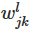来表示(l-1)层第k节点到l层第j节点的权重w
例如：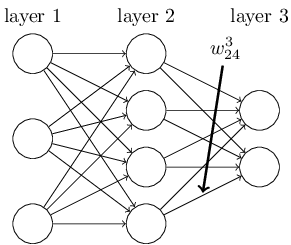我们用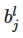来表示l层第j节点的偏差b，用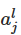来表示l层j节点所受到的总影响。
例如：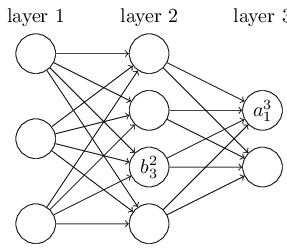可以分析得出整个神经网络正向是个递归推进的过程，公式如下：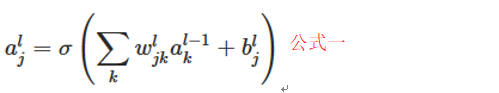其中σ是转换函数，在手写识别案例中σ就是sigmoid函数。
公式用矩阵简化后为：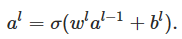用再对公式进行一次简化：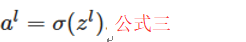整个网络输出的误差计算公式：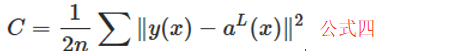这里需要注意前面用的都是小写的l，对应着具体的l层节点的运算；最终误差这里用的是大写的L，对应着整个神经网络的总体的运算。

以上都是铺垫，下面我们来真正看一下一旦我们对神经网络中的某个节点做出改变会如何影响到整个网络。
如图对l层第j节点有个小恶魔做了微小的变动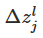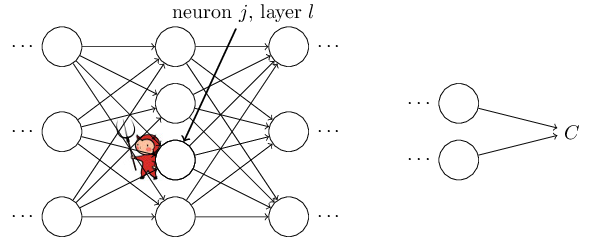改变后对该节点的产出变为：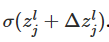，对α的的影响是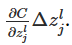假设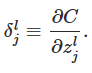套用公式三后结果为：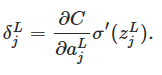前面介绍过L大小写的区别了，小恶魔对整个网络引起的差异速率为：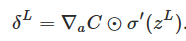对公式四求导带入上面公式得：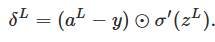PS：这是很重要的一步，因为万事开头难，对于反向传播来说整个网络output层反倒是修正时的最原始的input，到目前为止我们已经有了这个“input”的计算公式了，下面要解决的就是如何将其一点点往真正的input方向传递。

上面是站在整个网络上宏观的来分析对最终的输出造成的影响，下面我们聚焦到小恶魔只对后面一层(也就是l+1层的影响速率)
根据公式二推导出：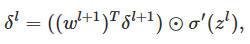PS：这是另一个里程碑式的成功，因为我们具备了从l+1层计算l层误差率的方式。

有了上面2个ps的理论，我们反向传播LàL-1àL-2à……l+1àlàl-1à….这条路就彻底打通了。

我们上面的小恶魔改动的是，最终我们产出的是每层的权重和偏差，再根据公式二求偏导：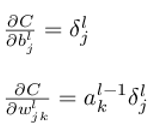这两个结果很容易推导的过来，但是给我们的启发是在求偏差还好通过结果数据可以计算的出来，但是在求每层权重的时候需要用到本节点的输入（也就是上一个节点的输出），所以我们在正向的运算时就需要把这些数据维护起来，否则无法完成反向传播的过程。

于是理解了上面的推导过程我们在处理反向传播时就有了一套很成熟的方法论了：
第一步：
将原始出入x作为α1的输入

第二步：
利用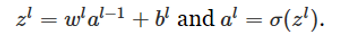正向运算记得维护每层的输出。

第三步：
利用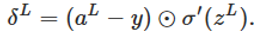求网络总输出的误差率

其实这么写是为了方便理解，因为我们这里的求误差的方式是公式四，如果不是用欧氏距离来求误差需要修改等号右边第一个括号内的内容。

第四步：
利用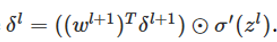从后向前求每一层的误差率

第五步：
利用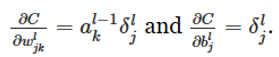求每一层的权重和偏差

第六步：
设置好步长和循环次数不断调优。

有了本篇的理论基础再回头看深度学习数学基础—反向传播求导过程和代码应该如虎添翼了吧。


展开全文神经网络 深度学习 算法
• ## 反向传播算法

千次阅读 2019-04-22 12:45:42
反向传播算法 微信公众号：幼儿园的学霸 个人的学习笔记，关于OpenCV,关于机器学习, …。问题或建议，请公众号留言; 在上一篇文章中对神经网络模型的前向...由于理解上可能有偏差，如果阅读上吃力的话也可以粗略...
反向传播算法

微信公众号：幼儿园的学霸
个人的学习笔记，关于OpenCV,关于机器学习, …。问题或建议，请公众号留言;

在上一篇文章中对神经网络模型的前向传播算法做了总结，这里更进一步，对神经网络的反向传播(Back Propagation，BP)算法的过程进行总结、数学推导。这一篇涉及的数学知识比较多，主要涉及的就是一个偏微分链式求导法则。由于理解上可能有偏差，如果阅读上吃力的话也可以粗略的浏览，大概了解反向传播算法即可。但是，学习这些数学知识可以帮助我们更深入的理解神经网络。
目录
文章目录反向传播算法目录反向传播算法要解决的问题损失函数/代价函数梯度下降反向传播损失函数的两个假设Hadamard积-⊙反向传播算法的四个基本方程及证明方程一：输出层的误差方程二：用当前层的误差表示下一层的误差方程三：代价函数与偏置的关系方程四：代价函数与权重的关系总结反向传播算法实现(神经网络训练流程)参考链接
反向传播算法要解决的问题
损失函数/代价函数
神经网络的作用就是我们预先给它大量的数据(包含输入和输出)来进行训练，训练完成后，我们希望它对于将来的真实环境的输入也能给出一个令我们满意的输出。怎么找到合适的参数呢？
这里我们引入损失函数(或称代价函数、Loss函数)的概念。
假设有n组包含了输入和真实结果(或称期望结果、期望输出)的样本数据，对于每组输入，神经网络的输出结果
记为$a_i$,真实结果(期望结果)记为$y_i$。
使用数学工具中的MAE（Mean Absolute Error,平均绝对误差），可以非常直观地表达出输出结果和真实结果的偏差，因此我们可以用MAE来写出一个下面这样的Loss函数，记为C，Loss值越大、说明神经网络的输出结果越远离我们的期望。
$C=\frac{1}{n}\sum_{i=1}^{n}|a_i-y_i|$
也可以用MSE（Mean Squared Error，均方误差）作为损失函数，MSE能更好地评价数据的变化程度，简单地说因为平方了一下、偏差是会被放大的。
$C=\frac{1}{n}\sum_{i=1}^{n}|a_i-y_i|^2$
将Sigmoid神经元的表达式$f(x)=σ(wx+b)$代入上面的损失函数中，可以发现x（输入）是固定的，yi（期望结果）也是固定的，让我们感性地想象一下：实际上影响Loss的只有w和b，而最重要的任务也就是寻找w和b使得Loss最小。
再具象一点，其实对神经网络进行训练的目的就是为每个神经元找到最适合它的w和b的值，从而使得整个神经网络的输出最接近我们的期望。

NOTE:最常用的损失函数
在实际中，为了方便求导，一般使用如下的二次损失函数：
$C=\frac{1}{2n}\sum_{i=1}^{n}|a_i-y_i|^2$

梯度下降
根据上面的结论，损失C只和权重w及偏置b 有关，那么可以把C看成是一个关于w和b的函数，如下所示。
$\bm {C=f(w,b)}$
由于神经网络中有大量的权重w和偏置b，因此上式按照上一篇中的结论，按矩阵形式进行表示。
对于简单的情况，上面的函数可能是这样的：我们的目标是找到W和b使C最小，当然上面这张图很容易看出来合适的W和b在哪，但当面对更复杂的情况时、比如下图这样的，应该如何快速地找到C最小的点呢？这里我们引入梯度下降算法，原理很简单：把上图看作是一个丘陵地带，想象我们有一个球放在某个位置，让它“自然地向低处滚”，滚得越低，C就越小，我们就越高兴。
那么怎样使得它往低处滚呢？(注意这里要搬出全文中第一个比较烧脑的概念了)微分法则告诉我们，当w移动Δw、b移动Δb时，有：
$\bm {\Delta C \approx \frac{\partial C}{\partial w}\Delta w + \frac{\partial C}{\partial b}\Delta b}$
由于C表示的是损失，我们想让球往低处滚，当然是希望C不断变小，那ΔC应该恒为负，那么Δw、Δb应该如何取值呢？ 梯度下降法是这么设计的：
$\bm{ \Delta W = -\eta \frac{\partial C}{\partial w} } \\ \bm{ \Delta b = -\eta \frac{\partial C}{\partial b} }$
可以看出如此取值可以使ΔC恒为负，其中的η称为学习率。
那么现在问题变成了∂C/∂w、∂C/∂b，即 C对w 和 C对b 的偏导,这就是反向传播算法所要解决的问题——
快速求解∂C/∂w、∂C/∂b，从而算出Δw和Δb，使得ΔC恒为负、即使得Loss越来越小。

NOTE:用了反向传播算法的多层感知机(神经网络)–也就是这篇文章讲的神经网络–也就叫作BP神经网络

反向传播
损失函数的两个假设
反向传播算法是为了计算损失函数的偏导数∂C/∂w、∂C/∂b，为了使算法可行，需要对损失函数的形式作两个假设。在介绍这些假设之前，先来看一个最常见的二次损失函数：
$\bm{ C=\frac{1}{2n} \sum_x ||y(x)-a^L(x)||^2} \quad \text(1)$
其中，n是训练样本总数，对所有训练样本损失求平均，y(x)是输入为x时对应的真实的输出，而L表示神经网络的层数，也就是说$a^L(x)$表示的是输入为x时，神经网络最后一层输出层的输出，也就是神经网络的输出。

第一个假设：
所有训练样本总的损失函数可以表示为单个样本损失函数和的平均值，即：$C=\frac{1}{n}\sum_x C_x$.我们很容易可以验证这个假设对于二次损失函数成立，$C_x=\frac{1}{2}||y-a^L||^2$。这个假设其实大部分时候都是成立，除了对于少数比较另类的损失函数，不过本文并不涉及。
我们需要这个假设的原因是因为反向传播算法实际是对于单个样本计算偏导数$\frac{\partial C_x}{\partial w} \text{和}\frac{\partial C_x}{\partial b}$,随后再通过对这些单样本的偏导数求平均作为$\frac{\partial C}{\partial w} \text{和}\frac{\partial C}{\partial b}$。事实上，在对w和b求偏导的时候，我们将输入x当作是固定值，所以方便起见，暂时将Cx写作C,后面再写回来。

第二个假设：
损失函数可以表示成神经网络输出的函数，即$C=C(a^L)$例如，二次损失函数就满足这样的假设，因为对于一个训练样本x来说，有：
$C=\frac{1}{2}||y-a^L||^2 = \frac{1}{2}\sum_j(y_j - a^L_j)^2$
这样就表示成了输出的函数，因为对于一个输入x来说，它实际正确的输出y是个固定值，并不是我们可以修改的变量。我们可以改变的只能是通过改变weights和biases来改变神经网络的输出$a^L$从而影响到损失函数的值。

Hadamard积-⊙
反向传播算法基于一些常见的线性代数操作：向量的相加，向量与矩阵的积等等。其中有一种操作不是很常见，这里简单介绍一下。假设s和t是两个相同维度的向量，我们使用s⊙t定义两个向量中对应分量相乘的操作，即$(s \odot t)_j = s_jt_j$例如：
$\left[ \begin{matrix} 1 \\ 2 \end{matrix} \right] \odot \left[ \begin{matrix} 3 \\ 4 \end{matrix} \right] = \left[ \begin{matrix} 1*3 \\ 2*4 \end{matrix} \right] = \left[ \begin{matrix} 3 \\ 8 \end{matrix} \right]$
这样的乘法操作被称为Hadamard积或Schur积。
反向传播算法的四个基本方程及证明
反向传播算法是关于理解改变weights和biases是如何改变损失函数C，也就是计算$\frac{\partial C}{\partial w^l_{jk}}\text{和}\frac{\partial C}{\partial b^l_{j}}$。在介绍如何计算这些偏导数之前，先引入一个中间变量$\delta^l_j$,称其为第l层第j个神经元的误差。反向传播算法会先计算这个中间变量，随后再将其与需要的偏导数关联起来。其定义为
$\delta^l_j = \frac{\partial C}{\partial z^l_j} \quad \text(2)$
反向传播算法将会对每一层l计算$\delta^l$,然后再得到对应的$\frac{\partial C}{\partial w^l_{jk}} \text{和} \frac{\partial C}{\partial b^l_j}$。
接下来就要介绍反向传播算法的四个方程了，同时将给出这些方程的简单证明。
方程一：输出层的误差
$\delta^L_j = \frac{\partial C}{\partial a^L_j} \sigma{}'(z^L_j) \quad \text(BP1)$
证明：
$\because \delta^L_j = \frac{\partial C}{\partial z^L_j}$
应用链式法则，我们可以就输出激活值的偏导数的形式重新表示上面的偏导数：
$\delta^L_j = \sum_k \frac{\partial C}{\partial a^L_k} \frac{\partial a^L_k}{\partial z^L_k}$
这里的求和是在输出层的所有神经元k上运行的，当然，第$k^{th}$个神经元的输出激活值$a^L_k$只依赖于当$k=j$时第$j^{th}$个神经元的带劝输入$z^L_j$。所以，当$k \neq j$时:
$\frac{\partial a^L_k}{\partial z^L_j} = 0$
则上一个方程可以简化为：
\begin{aligned} \delta^L_j = \frac{\partial C}{\partial z^L_j}\\ & =\frac{\partial C}{\partial a^L_j} \frac{\partial a^L_j}{\partial z^L_j} \\ & = \frac{\partial C}{\partial z^L_j} \frac{\partial \sigma(z^L_j)}{\partial z^L_j}\\ & =\frac{\partial C}{\partial a^L_j} \sigma{}'(z^L_j) \end{aligned}
注意到这个公式的每个部分都不难计算得到，$z^L_j\text{和} \sigma{}'(z^L_j)$在计算神经网络输出的时候可以得到，左边的部分在确定了损失函数的形式之后也可以计算得到。
公式BP1是针对输出层上的某一个神经元而言的，为了方便反向传播计算，可以将其改写为矩阵形式：
$\delta^L = \triangledown_a C \odot \sigma{}'(z^L) \quad \text(BP1a)$
对于这里的二次损失函数，有：
$\triangledown_a C = \triangledown_a(\frac{1}{2}\sum_j(y_j-a^L_j)^2) =a^L-y$
注意这里求导的过程只是对当前分量j求导，其余的分量就为0，于是有：
$\delta^L = (a^L-y) \odot \sigma{}'(z^L)$
方程二：用当前层的误差表示下一层的误差
$\delta^l = ((w^{l+1})^T)\delta^{l+1} \odot \sigma{}'(z^l) \quad \text(BP2)$
这个方程虽然看上去比较复杂，但是每个部分都有很明确的解释。假设我们知道l+1层的error：$\delta^{l+1}$，当同这一层的权重矩阵相乘的时候就类似于将这个error传到上一层，最后再利用Hadamard积得到l层的error。
证明：
应用链式法则：
\begin{aligned} \delta^l_j = \frac{\partial C}{\partial z^l_j} \\ & = \sum_k \frac{\partial C}{\partial z^{l+1}_k} \frac{\partial z^{l+1}_k}{\partial z^l_j} \\ & = \sum_k \delta^{l+1}_k \frac{\partial z^{l+1}_k}{\partial z^l_j} \\ & = \sum_k \frac{\partial z^{l+1}_k}{\partial z^l_j}\delta^{l+1}_k \end{aligned} \quad \text(3)
这里最后一行交换了右边的两项，并用$\delta^{l+1}_k$的定义代入。为了对最后一行的第一项求值，注意：
$z^{l+1}_k = \sum_j w^{l+1}_{kj}a^l_j+b^{l+1}_k = \sum_j w^{l+1}_{kj} \sigma(z^l_j) +b^{l+1}_k$
对上式做微分，得到：
$\frac{\partial z^{l+1}_k}{\partial z^l_j} = w^{l+1}_{kj} \sigma{}'(z^l_j)$
将上式代入式(3),可以得到：
$\delta^l_j = \sum_k w^{l+1}_{kj} \delta^{l+1}_k \sigma{}'(z^l_j)$
这正是以分量形式写的(BP2)

NOTE:
仔细观察上面公式中的$w^{l+1}_{kj}$会发现其中的j与k的顺序与w的原始定义中的顺序发生了对调，在原始定义中，第j个神经元的权重应是$w^{l+1}_{jk}$这样的形式，这可以理解成矩阵形式中转置的原因

通过组合(BP1)和(BP2)，我们可以计算任何层的误差$\delta^l$。首先使用(BP1)计算$\delta^l$，然后应用方程(BP2)来计算$\delta^{l-1}$,然后再次利用方程(BP2)来计算$\delta^{l-2}$，如此一步一步地反向传播完整个网络。
方程三：代价函数与偏置的关系
$\frac{\partial C}{\partial b^l_j} = \delta^l_j$
这其实是，误差$\delta^l_j$和偏导数值$\partial C / \partial b^l_j$完全一致。这是很好的性质，因为(BP1)和(BP2)已经告诉我们如何计算$\delta^l_j$。所以就可以将(BP3)简记为：
$\frac{\partial C}{\partial b} = \delta$
其中δ和偏置b都是针对同一个神经元。

NOTE：(BP3)的矩阵形式为：
$\frac{\partial C}{\partial b^l} = \delta^l$

证明：
根据链式法则展开：
\begin{aligned} \frac{\partial C}{\partial b^l_j} = \sum_k \frac{\partial C}{\partial z^l_k} \frac{\partial z^l_k}{\partial b^l_j} \\ & = \sum_k \delta^l_k \frac{\partial (\sum_j w^l_{kj}a^{l-1}_k+b^l_k)}{\partial b^l_j} \end{aligned}
因为只有当$k=j$的时候上式最后一项的右边部分才为1，而当$k\neq j$时，
$\frac{\partial (w^l_{kj}a^{l-1}_k+b^l_k)}{\partial b^l_j} = 0$
所以上式简化为
\begin{aligned} \frac{\partial C}{\partial b^l_j} = \sum_k \frac{\partial C}{\partial z^l_k} \frac{\partial z^l_k}{\partial b^l_j} \\ & = \sum_k \delta^l_k \frac{\partial (\sum_j w^l_{kj}a^{l-1}_k+b^l_k)}{\partial b^l_j} \\ & = \delta^l_j * 1 \\ & = \delta^l_j \end{aligned}
方程(BP3)得证！
方程四：代价函数与权重的关系
$\frac{\partial C}{\partial w^l_{jk}} = a^{l-1}_k\delta^l_j \quad \text(BP4)$
这个方程说明，当我们要计算某两个神经元链接的权重对损失函数影响的时候，可以先计算上一层的$a^{l-1}_k$和下一层的$\delta^l_j$。而这两个值我们根据先前的知识已经知道怎么计算了。这个方程也可以被写为：
$\frac{\partial C}{\partial w} = a_{in}\delta_{out} \quad \text(4)$
从公式(4)可以看出来，当$a_{in} \approx 0$的时候，$\frac{\partial C}{\partial w}$也会很小，那样这个权重的学习就会很慢，意味着在梯度下降的学习过程中，这个权重不会发生大的变化，换句话说就是，输出小的神经元的权重学习也慢。
证明：
额，这个证明和(BP3)的证明过程是一样的。
为了避免误解，这里的下表稍微改下，
\begin{aligned} \frac{\partial C}{\partial bw^l_{jk}} = \sum_a \frac{\partial C}{\partial z^l_a} \frac{\partial z^a_k}{\partial bw^l_{jk}} \\ & = \sum_a \delta^l_k \frac{\partial (\sum_j w^l_{kj}a^{l-1}_k+b^l_k)}{\partial b^l_j} \end{aligned}
很显然，
$\frac{\partial b^l_a}{\partial w^l_{jk}} = 0$
,而另一部分只有在$a=j,b=k$时才不会0，此时就可以去掉求和符号得到：
$\frac{\partial C}{\partial w^l_{jk}} = a^{l-1}_k\delta^l_j$
方程(BP4)得证！

NOTE：方程(BP4)的矩阵形式为：
$\frac{\partial C}{\partial w^l} = \delta^l (a^{l-1})^T$

总结
通过上面推导，可以发现在经过一次正向传播之后，可以通过输出层的误差、快速求解出C对每个w和b的偏导，即∂C/∂w、∂C/∂b，再对每个w和b加上Δw、Δb，从而使得“球往下滚”，C、即Loss越来越小，神经网络在朝着我们期望的方向进行调整。
反向传播算法实现(神经网络训练流程)
基于上面的知识，我们现在可以总结出训练一个神经网络的全流程：
1.初始化神经网络.(1)设置输入层的输出为原始的输入x，即：$a^1=x$;
(2)对每个神经元的w和b赋予随机值
2.对神经网络进行前向传播计算，得到输出层各个神经元的带权输入和激活输出:$z^l=w^la^{l-1}+b^l \text{和}a^l=\sigma(z^l)$
3.求出输出层的误差$\delta^L = \triangledown_aC \odot \sigma{}'(z^L)$
4.通过反向传播算法，向后求出每一层(的每个神经元)的误差:$\delta^l = ((w^{l+1})^T\delta^{l+1}) \odot \sigma{}'(z^L)$
5.通过误差可以计算各个梯度:$\frac{\partial C}{\partial w^l_{jk}} = a^{l-1}_k\text{和} \frac{\partial C}{\partial b^l_j} = \delta^l_j$  得出每个神经元的∂C/∂w、∂C/∂b，再乘上负的学习率(-η)，就得到了Δw、Δb，将每个神经元的w和b更新为 w+Δw、b+Δb；
完成训练之后，一般情况下我们都能得到一个损失比较小的神经网络。
从算法的流程也可以明白为什么它被称为反向传播算法，因为我们是从最后一层的$\delta^L$开始反向计算前面的$\delta^l$的。因为损失函数是关于神经网络输出的函数，所以为了得到损失函数关于前面层的参数的梯度就需要不断的应用求导链式法则，一层层向前推导得到我们需要的关系的表达式。
参考链接
1. Neural Networks and Deep Learning,开源，有中文版，值得一看。

下面的是我的公众号二维码图片，欢迎关注。展开全文• 反向传播算法广泛用在机器学习、人工智能等领域，目的在于将各个结点的权值w和偏差值b等参数调节到最优状态（最优状态：使训练数据的平均效果达到最好）。反向传播算法与前向传播算法都是神经网络的经典算法，正如...


反向传播算法概念
反向传播算法细节
代码细节
代码分析

反向传播算法概念

反向传播算法广泛用在机器学习、人工智能等领域，目的在于将各个结点的权值w和偏差值b等参数调节到最优状态（最优状态：使训练数据的平均效果达到最好）。反向传播算法与前向传播算法都是神经网络的经典算法，正如它们的名字一样。反向传播算法是从输出层一直传递到输入层，传递的过程主要做的动作是微调权值和偏差值；前向传播主要确定各层结点的输入与输出，最后计算输出层上所有结点的输出值（实际计算值）以及误差值（误差值：输出目标值与实际计算值之间的距离，具体公式典型的有：求差和欧式距离等）。

反向传播算法细节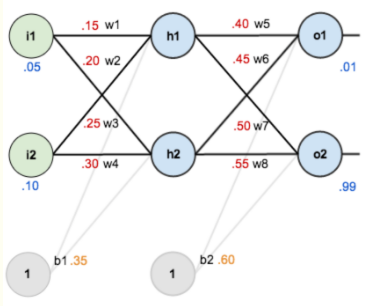图片来自 http://blog.csdn.net/mao_xiao_feng/article/details/53048213

反向传播算法主要涉及到的数学原理是：链式求导，它是该算法的核心和精髓所在，掌握这个方法，就基本上学会了反向传播算法。想要真正掌握这个算法，就需要用代码实现一下了，但是它与神经网络耦合严重，所以需要掌握神经网络细节，有一定的挑战性，但想要成功的你一定可以办到 -_-||

1. 前向传播算法
前向传播主要确定各层结点的输入与输出，最后计算输出层上所有结点的输出值（实际计算值）以及误差值（误差值：输出目标值与实际计算值之间的距离，具体公式典型的有：求差和欧式距离等）。

上图中，从左到右依次是输入层、隐藏层（可以为多层，本例为一层）和输出层。i1和i2是输入层结点，h1和h2是隐藏层结点，o1和o2是输出层结点。前向传播主要确定各结点的值和全局误差（也称全局损失，由全局损失函数确定）和输出层各个结点的误差（局部误差）（也称局部损失，由局部损失函数确定）。各权重和偏差的初始值在图中已经给出，下面是求解过程（该求解过程为一次前向传播过程，对应一个实例的训练过程）：

1.1首先，涉及到的变量有下面16个：

i1 = 0.05；h1 = 待求；o1 = 待求；w1 = 0.15；w3 = 0.25；w5 = 0.40；w7 = 0.50；b1 = 0.35
i2 = 0.10；h2 = 待求；o2 = 待求；w2 = 0.20；w4 = 0.30；w6 = 0.45；w8 = 0.55；b2 = 0.60

前向过程主要求出h1、h2、o1和o2的输出值（输出值：激活函数函数值）

激活函数: （懂激活函数可以跳过此处。）把结点的输入值作为自变量的函数，激活函数的函数值为该结点的输出值。激活函数来源于感受器的概念，一个感受器代表一个结点的过滤器，主要目的是矫正结点的值，使之在固定的范围内。比如：0-1。也就是激活函数的值域有固定的范围，比如典型的激活函数：sigmoid函数 y=11+e−x$y=\frac{1}{1+{e}^{-}x}$y=\frac{1}{1 + e^  -x} 它的值域为（0，1），所以可以保证每个结点的输出值都在0-1之间。

1.2然后计算待求值：

h1计算过程：
h1的输入值neth1
neth1=i1w1+i2w2+b1$net{h}_{1}={i}_{1}{w}_{1}+{i}_{2}{w}_{2}+{b}_{1}$net h_1 = i_1 w_1 + i_2w_2 + b_1
h1的输出值h1
h1=func(neth1)${h}_{1}=func\left(net{h}_{1}\right)$h_1 = func(neth_1)
（func为激活函数，如果为sigmoid时）
h1=11+e−neth1${h}_{1}=\frac{1}{1+{e}^{-}net{h}_{1}}$h_1 = \frac{1}{1 + e^-neth_1}

h2计算过程：
h2的输入值neth2
neth2=i1w3+i2w4+b1$net{h}_{2}={i}_{1}{w}_{3}+{i}_{2}{w}_{4}+{b}_{1}$net h_2 = i_1 w_3 + i_2w_4 + b_1
h2的输出值h2
h2=func(neth2)${h}_{2}=func\left(net{h}_{2}\right)$h_2 = func(neth_2)
（func为激活函数，如果为sigmoid时）
h1=11+e−neth2${h}_{1}=\frac{1}{1+{e}^{-}net{h}_{2}}$h_1 = \frac{1}{1 + e^-neth_2}
o1和o2计算过程类似，这里省略。
1.3最后求全局误差值E和局部误差值Ei
局部误差函数（误差函数就是神经网络中的损失函数，误差函数是数学术语，损失函数是机器学习领域术语）的选择有多种，如果是误差平方和，则：Ei=12(oi−oi目标值)2${E}_{i}=\frac{1}{2}\left({o}_{i}-{o}_{i}目标值{\right)}^{2}$E_i = \frac{1}{2}(o_i - o_i目标值)^2
全局误差函数
E=∑inEi$E=\sum _{i}^{n}{E}_{i}$E = \sum_i^n E_i

这里的误差函数是神经网络调优的一个参数

2. 反向传播算法
如果神经网络没有反向传播，只有前向传播，则前向传播从第二个实例进入神经网络，开始调节权重值和偏差值。只有前向传播过程的人工神经网络也称为序贯神经网络模型。

反向传播算法完成对权重值和偏差值的调节，主要用到链式求导z=y$z=y$z = y ,y=a+b$y=a+b$y = a + b, 则z对a求导为：z′(a)=z′(y)y′(a)$z\mathrm{\prime }\left(a\right)=z\mathrm{\prime }\left(y\right)y\mathrm{\prime }\left(a\right)$z\prime(a) = z\prime(y)y\prime(a)

具体求解过程（该求解过程为一次反向传播过程，对应一个实例的训练过程）：
1输出层到隐藏层
w5

w5=w5−α⋅∂E∂w5${w}_{5}={w}_{5}-\alpha \cdot \frac{\mathrm{\partial }E}{\mathrm{\partial }{w}_{5}}$w_5 = w_5 - \alpha\cdot\frac{\partial E}{\partial w_5}
∂E∂w5=∂E∂o1⋅∂o1∂neto1⋅∂neto1∂w5$\frac{\mathrm{\partial }E}{\mathrm{\partial }{w}_{5}}=\frac{\mathrm{\partial }E}{\mathrm{\partial }{o}_{1}}\cdot \frac{\mathrm{\partial }{o}_{1}}{\mathrm{\partial }net{o}_{1}}\cdot \frac{\mathrm{\partial }net{o}_{1}}{\mathrm{\partial }{w}_{5}}$\frac{\partial E}{\partial w_5} = \frac{\partial E}{\partial o_1}\cdot\frac{\partial o_1}{\partial neto_1}\cdot\frac{\partial neto_1}{\partial w_5}
alpha为正数，如：0.5
这里解释一下为什么用减而不用加：偏导值为正数，表示w5与E正相关，也就是w5越大，E越大。所以此时减取一个正数，w5减少了，也就是全局误差E减少了。所以理论意义与物理意义符合逻辑。偏导值为负数时道理一样。全局误差E减少正是我们的目标。

w6

w6=w6−α⋅∂E∂w6${w}_{6}={w}_{6}-\alpha \cdot \frac{\mathrm{\partial }E}{\mathrm{\partial }{w}_{6}}$w_6 = w_6 - \alpha\cdot\frac{\partial E}{\partial w_6}
∂E∂w6=∂E∂o1⋅∂o1∂neto1⋅∂neto1∂w6$\frac{\mathrm{\partial }E}{\mathrm{\partial }{w}_{6}}=\frac{\mathrm{\partial }E}{\mathrm{\partial }{o}_{1}}\cdot \frac{\mathrm{\partial }{o}_{1}}{\mathrm{\partial }net{o}_{1}}\cdot \frac{\mathrm{\partial }net{o}_{1}}{\mathrm{\partial }{w}_{6}}$\frac{\partial E}{\partial w_6} = \frac{\partial E}{\partial o_1}\cdot\frac{\partial o_1}{\partial neto_1}\cdot\frac{\partial neto_1}{\partial w_6}

w7

w7=w7−α⋅∂E∂w7${w}_{7}={w}_{7}-\alpha \cdot \frac{\mathrm{\partial }E}{\mathrm{\partial }{w}_{7}}$w_7 = w_7 - \alpha\cdot\frac{\partial E}{\partial w_7}
∂E∂w7=∂E∂o2⋅∂o2∂neto2⋅∂neto2∂w7$\frac{\mathrm{\partial }E}{\mathrm{\partial }{w}_{7}}=\frac{\mathrm{\partial }E}{\mathrm{\partial }{o}_{2}}\cdot \frac{\mathrm{\partial }{o}_{2}}{\mathrm{\partial }net{o}_{2}}\cdot \frac{\mathrm{\partial }net{o}_{2}}{\mathrm{\partial }{w}_{7}}$\frac{\partial E}{\partial w_7} = \frac{\partial E}{\partial o_2}\cdot\frac{\partial o_2}{\partial neto_2}\cdot\frac{\partial neto_2}{\partial w_7}

w8

w8=w8−α⋅∂E∂w8${w}_{8}={w}_{8}-\alpha \cdot \frac{\mathrm{\partial }E}{\mathrm{\partial }{w}_{8}}$w_8 = w_8 - \alpha\cdot\frac{\partial E}{\partial w_8}
∂E∂w8=∂E∂o2⋅∂o2∂neto2⋅∂neto2∂w8$\frac{\mathrm{\partial }E}{\mathrm{\partial }{w}_{8}}=\frac{\mathrm{\partial }E}{\mathrm{\partial }{o}_{2}}\cdot \frac{\mathrm{\partial }{o}_{2}}{\mathrm{\partial }net{o}_{2}}\cdot \frac{\mathrm{\partial }net{o}_{2}}{\mathrm{\partial }{w}_{8}}$\frac{\partial E}{\partial w_8} = \frac{\partial E}{\partial o_2}\cdot\frac{\partial o_2}{\partial neto_2}\cdot\frac{\partial neto_2}{\partial w_8}

2隐藏层到输入层
w1

w1=w1−α⋅∂E∂w1${w}_{1}={w}_{1}-\alpha \cdot \frac{\mathrm{\partial }E}{\mathrm{\partial }{w}_{1}}$w_1 = w_1 - \alpha\cdot\frac{\partial E}{\partial w_1}
∂E∂w1=∂E∂h1⋅∂h1∂neth1⋅∂neth1∂w1$\frac{\mathrm{\partial }E}{\mathrm{\partial }{w}_{1}}=\frac{\mathrm{\partial }E}{\mathrm{\partial }{h}_{1}}\cdot \frac{\mathrm{\partial }{h}_{1}}{\mathrm{\partial }net{h}_{1}}\cdot \frac{\mathrm{\partial }net{h}_{1}}{\mathrm{\partial }{w}_{1}}$\frac{\partial E}{\partial w_1} = \frac{\partial E}{\partial h_1}\cdot\frac{\partial h_1}{\partial neth_1}\cdot\frac{\partial neth_1}{\partial w_1}
∂E∂h1=∂E1∂h1+∂E2∂h1$\frac{\mathrm{\partial }E}{\mathrm{\partial }{h}_{1}}=\frac{\mathrm{\partial }{E}_{1}}{\mathrm{\partial }{h}_{1}}+\frac{\mathrm{\partial }{E}_{2}}{\mathrm{\partial }{h}_{1}}$\frac{\partial E}{\partial h_1} = \frac{\partial E_1}{\partial h_1}+\frac{\partial E_2}{\partial h_1}

w2

w2=w2−α⋅∂E∂w2${w}_{2}={w}_{2}-\alpha \cdot \frac{\mathrm{\partial }E}{\mathrm{\partial }{w}_{2}}$w_2 = w_2 - \alpha\cdot\frac{\partial E}{\partial w_2}
∂E∂w2=∂E∂h1⋅∂h1∂neth1⋅∂neth1∂w1$\frac{\mathrm{\partial }E}{\mathrm{\partial }{w}_{2}}=\frac{\mathrm{\partial }E}{\mathrm{\partial }{h}_{1}}\cdot \frac{\mathrm{\partial }{h}_{1}}{\mathrm{\partial }net{h}_{1}}\cdot \frac{\mathrm{\partial }net{h}_{1}}{\mathrm{\partial }{w}_{1}}$\frac{\partial E}{\partial w_2} = \frac{\partial E}{\partial h_1}\cdot\frac{\partial h_1}{\partial neth_1}\cdot\frac{\partial neth_1}{\partial w_1}
∂E∂h1=∂E1∂h1+∂E2∂h1$\frac{\mathrm{\partial }E}{\mathrm{\partial }{h}_{1}}=\frac{\mathrm{\partial }{E}_{1}}{\mathrm{\partial }{h}_{1}}+\frac{\mathrm{\partial }{E}_{2}}{\mathrm{\partial }{h}_{1}}$\frac{\partial E}{\partial h_1} = \frac{\partial E_1}{\partial h_1}+\frac{\partial E_2}{\partial h_1}

w3

w3=w3−α⋅∂E∂w3${w}_{3}={w}_{3}-\alpha \cdot \frac{\mathrm{\partial }E}{\mathrm{\partial }{w}_{3}}$w_3 = w_3 - \alpha\cdot\frac{\partial E}{\partial w_3}
∂E∂w3=∂E∂h2⋅∂h2∂neth2⋅∂neth2∂w3$\frac{\mathrm{\partial }E}{\mathrm{\partial }{w}_{3}}=\frac{\mathrm{\partial }E}{\mathrm{\partial }{h}_{2}}\cdot \frac{\mathrm{\partial }{h}_{2}}{\mathrm{\partial }net{h}_{2}}\cdot \frac{\mathrm{\partial }net{h}_{2}}{\mathrm{\partial }{w}_{3}}$\frac{\partial E}{\partial w_3} = \frac{\partial E}{\partial h_2}\cdot\frac{\partial h_2}{\partial neth_2}\cdot\frac{\partial neth_2}{\partial w_3}
∂E∂h2=∂E1∂h2+∂E2∂h2$\frac{\mathrm{\partial }E}{\mathrm{\partial }{h}_{2}}=\frac{\mathrm{\partial }{E}_{1}}{\mathrm{\partial }{h}_{2}}+\frac{\mathrm{\partial }{E}_{2}}{\mathrm{\partial }{h}_{2}}$\frac{\partial E}{\partial h_2} = \frac{\partial E_1}{\partial h_2}+\frac{\partial E_2}{\partial h_2}

w4

w4=w4−α⋅∂E∂w4${w}_{4}={w}_{4}-\alpha \cdot \frac{\mathrm{\partial }E}{\mathrm{\partial }{w}_{4}}$w_4 = w_4 - \alpha\cdot\frac{\partial E}{\partial w_4}
∂E∂w4=∂E∂h2⋅∂h2∂neth2⋅∂neth2∂w4$\frac{\mathrm{\partial }E}{\mathrm{\partial }{w}_{4}}=\frac{\mathrm{\partial }E}{\mathrm{\partial }{h}_{2}}\cdot \frac{\mathrm{\partial }{h}_{2}}{\mathrm{\partial }net{h}_{2}}\cdot \frac{\mathrm{\partial }net{h}_{2}}{\mathrm{\partial }{w}_{4}}$\frac{\partial E}{\partial w_4} = \frac{\partial E}{\partial h_2}\cdot\frac{\partial h_2}{\partial neth_2}\cdot\frac{\partial neth_2}{\partial w_4}
∂E∂h2=∂E1∂h2+∂E2∂h2$\frac{\mathrm{\partial }E}{\mathrm{\partial }{h}_{2}}=\frac{\mathrm{\partial }{E}_{1}}{\mathrm{\partial }{h}_{2}}+\frac{\mathrm{\partial }{E}_{2}}{\mathrm{\partial }{h}_{2}}$\frac{\partial E}{\partial h_2} = \frac{\partial E_1}{\partial h_2}+\frac{\partial E_2}{\partial h_2}
偏差值的计算与此类似，这里省略。

代码细节

java实现

    /**
* 前馈算法<hr>
* 默认激活函数tanh，若自定义激活函数则自行修改本函数
* @param layers 所有神经层
* @param weights 所有权重值
* @param bis 所有偏置值
* @return
*/
public static Map<Integer, List<List<Neural>>> forward(Layer[] layers, Map<Integer, double[][]> weights, List<Double> bis) {

Map<Integer, List<List<Neural>>> result = new HashMap<Integer, List<List<Neural>>>();
List<List<Neural>> inputs = new ArrayList<List<Neural>>();
List<List<Neural>> outputs = new ArrayList<List<Neural>>();
result.put(0, inputs);
result.put(1, outputs);
for (int i = 0; i < layers.length-1; i++) {
List<Neural> inputNeurals = new ArrayList<Neural>();
List<Neural> outputNeurals = new ArrayList<Neural>();
inputs.add(inputNeurals);
outputs.add(outputNeurals);
for (int j = 0; j < layers[i+1].getSize(); j++) {
inputNeurals.add(new Neural(0));
outputNeurals.add(new Neural(0));
}
}

for (int i = 0; i < layers.length; i++) {
if (i == 0) continue;
for (int j = 0; j < layers[i].getSize(); j++) {

double sum = 0;
for (int z = 0; z < layers[i-1].getSize(); z++) {
sum += layers[i-1].getNeurals().get(z).getValue() * weights.get(i-1)[z][j];
}
sum += bis.get(i-1);
inputs.get(i-1).get(j).setValue(sum);

sum = ActivationFunction.tanh(sum);
layers[i].getNeurals().get(j).setValue(sum);
outputs.get(i-1).get(j).setValue(sum);
}
}

return result;
}

代码分析
展开全文• 一旦我们有了这些偏导数，我们将通过一些常数 α的乘积和该数量相对于成本函数的偏导数来更新网络中的权重和偏差。这是流行的梯度下降算法。而偏导数给出了最大上升的方向。因此，关于反向传播算法，我们继续查看...
• 【一些基础概念】 误差反向传播（Error Back Propagation, BP）算法 1、BP算法的基本思想是：学习过程由信号的正向...第二步：加权后的输入求和，加上一个偏差b（绿色） 第三步：这个结果传递给一个激活函数f(x.
• 反向传播算法：计算输出层结果与真实值之间的偏差来进行逐层调节参数（逐层条件参数一般用到梯度下降算法） 首先我们得明白神经网络的参数训练是一个不断迭代的过程 当网络结构已知时，参数训练的过程如下： 通过...python TensorFlow
• BP(Backpropagation Algorithm，反向传播算法)在神经...文中如有理解偏差，请各位指正。 就反向传播的字面理解是将数据从后(输出)向前(输入)传递，数据的具体形式是代价函数对其超参数(权重(W)和偏置(b))的偏导数，...优化算法
• 在上一章中，我们看到了神经网络如何使用梯度下降算法来学习它们的权值和偏差。然而，在我们的解释中有一个缺口:我们没有讨论如何计算成本函数的梯度。在这一章中，我将解释一种计算这种梯度的快速算法，一种称为...
• 这个学生犯的错误就是，他的注意力出现了一定的偏差，他关注于挑别人毛病，以彰显自己的能耐和不一样，其实是一种反向的聪明！因为他真正需要关注的是书的内容，书的精神，书的价值观，而不是毛病！
• 我们学习了神经网络如何使用梯度下降算法来学习他们自身的权重和偏差。但是，这里还留下了一个问题：我们并没有讨论如何计算代价函数的梯度。这是很大的缺失！在本章，我们会解释计算这些梯度的快速算法，也就是反向...
• 优化算法基本上都是在反向传播算出梯度之后进行改进的，因为反向传播算法是一个递归形式，通过一层层的...那么如何根据一个网络的loss来更新参数，即如何更新网络节点中的权重w和偏差b的值？ 神经网络中的参数说明：...BP算法
• 通过时间反向传播 介绍循环神经网络中梯度的计算和存储方法，即通过时间反向传播（back-propagation through time...考虑一个简单的无偏差项的循环神经网络，且激活函数为恒等映射（ϕ(x)=x\phi(x)=xϕ(x)=x）。设时间python 神经网络 深度学习 算法
• 卷积层是卷积神经网络中非常重要的层，所以这里我们推导一下在卷积层的反向传播算法。 首先我们要思考一下卷积层的一些要素，要素很多包括权值、偏差等，但是我们只考虑权值和偏差，因为在训练过程中它们需要不断地...深度学习
• 改进了HS模型的假设条件,增加了反向投资者行为偏差的影响,建立了考虑卖空限制的动量效应和反向效应模型.在研究方法上,采用了不同于HS模型的递推公式法,清晰地描述了正、...
• 由于要更新参数,所以求 这玩意又等于L对w的偏导 这玩意又可以用链乘 ...右边那玩意用反向传播 就是知道预测的和实际的偏差距离来求 正向反向的数相乘可以凑到更新w权值需要的数,然后更新权值 ...
• 大多数人对好运气的理解可能会有些偏差，甚至是过于物质了，因为我们对它的关注点依然停留在客观世界。在我看来，好运气更多的是着落在个人的主观精神世界里，一旦拥有了这样的机缘，才能不断地边走边悟。 恰恰期货...
• 转载自链接：https://blog.csdn.net/baozi__/article/details/78307479计算过程现在我们有这样一个神经网络： 输入层有两个神经元i1和i2，隐藏层有两个神经元h1和h2，偏差都为b1，输出层有两个神经元o1和o2，偏差都...
• 1 设计反向题的目的被试对问卷的反应是存在偏差的。其中比较关键的有默许偏差，被试更倾向于同意所有题目或者作出积极的评价；社会赞许偏差，被试倾向于按照社会所期许的方式作出反应。由于反应偏差的存在，通过问卷...
• 一旦我们有了这些偏导数，我们将通过一些常数 α的乘积和该数量相对于成本函数的偏导数来更新网络中的权重和偏差。这是流行的梯度下降算法。而偏导数给出了最大上升的方向。因此，关于反向传播算法，我们继续查看...python 反传播
• 问题1，为何反向端和同相端的偏差这么多？ 问题2，运放的输出腿应该是低阻，为何加万用表（万用表的直流电压档用另外一个万用表测量阻值是10M）影响这么大呢？ 3.反思总结 1.经过测试，发现实际测量中，万用表...
• 反向传播算法工作原理在上一篇文章，我们看到了神经网络如何通过梯度下降算法学习，从而改变权重和偏差。但是，前面我们并没有讨论如何计算代价函数的梯度，这是一个很大的遗憾。这一篇文章，我们将介绍一种称为反向...神经网络 深度学习 算法
• 前言 本节将介绍循环神经网络中梯度的计算和存储方法，即 通过时间反向传播（back-...简单起见，我们考虑一个无偏差项的循环神经网络，且激活函数为恒等映射（ϕ(x)=x\phi(x)=xϕ(x)=x）。设时间步 ttt 的输入为单样本神经网络 深度学习 人工智能 pytorch
• 我记得在之前有好几篇文章都说过，期货反向跟单本质上是一个管理导向型的交易策略。可能大家没有注意到，或者理解有所偏差，我觉得朋友们混淆了管理的概念。 管理是指对交易员情绪的管理，对他们交易数据处理再加工...
• 摘自《动手学习深度学习》 这里介绍循环神经网络中梯度的计算和存储方法 在深度学习理论：正向传播与反向传播中讲了正向传播和反向传播的计算过程，主要依据...考虑一个无偏差项的循环神经网络，且激活函数为恒等...
• 对于神经网络的训练过程而言，其反向传播算法是训练过程的核心，神经网络根据预测值y^y^与实际值yy的偏差从后向前来计算损失函数对于各个参数的梯度，从而利用梯度下降的方法来优化训练神经网络的各个参数。......